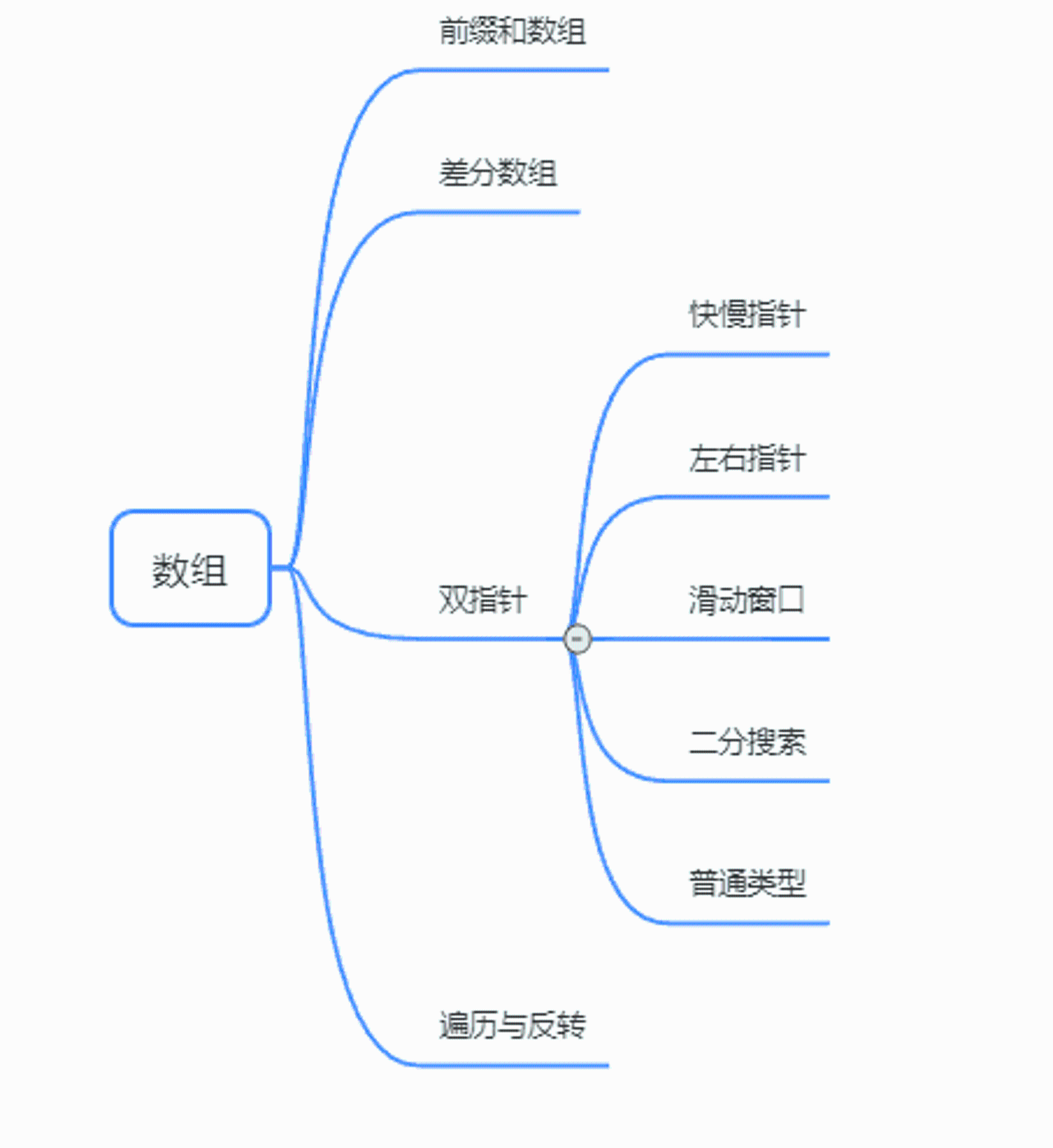xicheng

V1

2022/06/23阅读：15主题：默认主题

# 前言

上篇文章讲了前缀和前缀和数组，这篇文章开始讲查分数组。另外，数组有下图这些知识点与技巧。# 思路

场景：频繁对原始数组的某个区间的元素进⾏增减。原数组nums与差分数组diff如下图所示。

# 区间加法

leetcode第370题

直接在差分数组上做操作：diff[startIndex] = diff[startIndex] + inc， diff[endIndex + 1] = diff[endIndex + 1] - inc。
这就代表了原数组nums的startIndex到endIndex位置的元素都加上inc。
但当endIndex就是指向nums的最后一个元素时，就无需做diff[endIndex + 1] = diff[endIndex + 1] - inc操作了。

时间复杂度：O(n + m)，n是差分数组的长度，m是操作的次数。对于每一次操作都要处理一次差分数组，并最后对差分数组求前缀和。
空间复杂度：O(1)，注意返回值不计入空间复杂度。

``class Solution {   int[] getModifiedArray(int length, int[][] updates) {      int[] diff = new int[length];      for (int[] u : updates) {         diff[u] += u;         if (u < length - 1) {            diff[u + 1] -= u;         }      }      for (int i = 1; i < length; i++) {         diff[i] = diff[i - 1] + diff[i];      }      return diff;   }}``

# 航班预订统计

leetcode第1109题

思路与《区间加法》基本一致。但要注意操作数组中的下标是从1开始而不是0。

时间复杂度：O(n + m)，n是差分数组的长度，m是操作的次数。对于每一条预定记录都要处理一次差分数组，并最后对差分数组求前缀和。 　　空间复杂度：O(1)，注意返回值不计入空间复杂度。

``class Solution {    public int[] corpFlightBookings(int[][] bookings, int n) {        int[] diff = new int[n];        for (int[] b : bookings) {            diff[b - 1] += b;            if (b - 1 < n - 1) {                diff[b] -= b;            }        }        for (int i = 1; i < n; i++) {            diff[i] = diff[i - 1] + diff[i];        }        return diff;    }}``

# 拼车

leetcode第1094题

注意toi下标对应的含义为乘客在此处下车，那么差分数组需要在此处减去对应的乘客数。
在最后将差分数组转化为原数组进行判断是否超载时，必须要额外判断原数组第0项是否超载（这里很容易遗漏）。

时间复杂度：O(n + m)，n是差分数组的长度，m是操作的次数。对于每一次旅行都要处理一次差分数组，并最后对差分数组求前缀和。
空间复杂度：O(n)，需要额外空间存储查分数组。

``class Solution {    public boolean carPooling(int[][] trips, int capacity) {       //先找出所有乘客下车的站中的最大的那个站      int max = Stream.of(trips).max(Comparator.comparingInt(o -> o)).map(o -> o).get();      int len = max + 1;      int[] diff = new int[len];      for (int[] u : trips) {         diff[u] += u;         //注意，在哪个站站下车，差分数组就需要在对应的下表处做减法         if (u < len) {            diff[u] -= u;         }      }      //差分数组/元素组的第0项也要做判断      if (diff > capacity) {         return false;      }      for (int i = 1; i < len; i++) {         diff[i] = diff[i - 1] + diff[i];         if (diff[i] > capacity) {            return false;         }      }      return true;    }}``

# 结尾

通过三道差分数组算法题的练习，相信你学会了其中的精髓了。下一篇算法文章讲双指针之快慢指针。

感谢各位人才的点赞、收藏和评论，干货文章持续更新中，下篇文章再见！V1